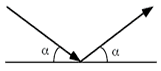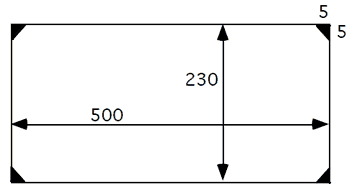## Problem S5: Follow the Bouncing Ball

Suppose you want to create a screen saver which has a ball bouncing around the edges. The action will continue until the ball hits a corner (like a pool table). At that point, the ball disappears off the screen and the screen goes black.Balls bounce off the walls according to the laws of physics. The angle of the ball as it approaches the wall (the angle of incidence) will equal the angle of the ball as it bounces away (the angle of reflection).

Write a program which will determine how many bounces it will take before the bouncing ball will be swallowed by a corner, if at all. The screen dimensions are in the range [100, 1000] units. Consider the ball as a point on the screen. The corner pockets are 5 units along each wall, and if a ball hits the wall at the edge of a pocket it will continue bouncing.

NOTE: The ball will always hit the right wall first.

A sample screen:### Input

Input consists of four integers on separate lines.

n - The width of the screen. (100 ≤ n ≤ 1000)
m - The height of the screen. (100 ≤ m ≤ 1000)
p - The initial position of the ball at the bottom of the screen. (5 ≤ p ≤ (n - 5))
q - The position of the ball when it bounces off the right wall. (5 ≤ q ≤ (m - 5))

### Output

The output consists of an integer which indicates the number of bounces the ball makes before it disappears in a corner. Count the hit off the right wall as the first bounce. It is possible that the ball will never bounce into a corner (assuming a frictionless surface), in this case, output should be 0. Note that when the ball hits a pocket is not counted as a bounce. You do not have to program the actual animation.

```300
200
200
100```

### Sample Output

`2`

Point Value: 10
Time Limit: 2.00s
Memory Limit: 16M

Problem Types: [Show]

Languages Allowed:
C++03, PAS, C, HASK, ASM, RUBY, PYTH2, JAVA, PHP, SCM, CAML, PERL, C#, C++11, PYTH3